Скачать презентацию 3 -8 Solving Equations Containing Fractions Warm Up

eee979c81b3dd07c7d8a8045e2ecd789.ppt

• Количество слайдов: 46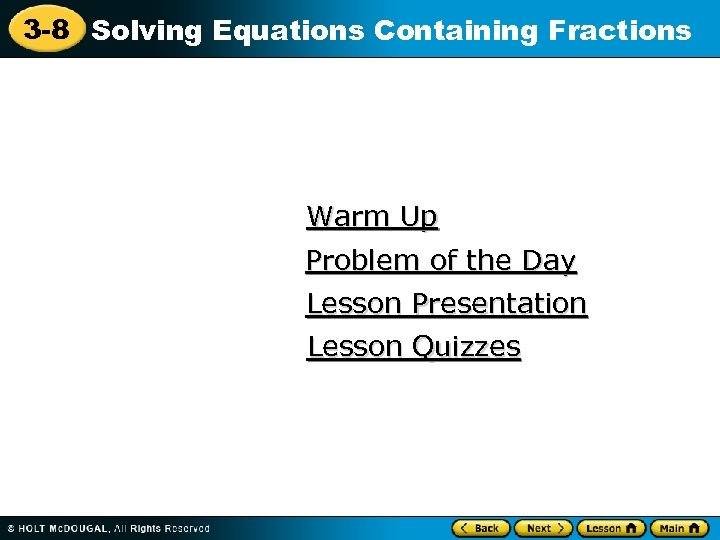3 -8 Solving Equations Containing Fractions Warm Up Problem of the Day Lesson Presentation Lesson Quizzes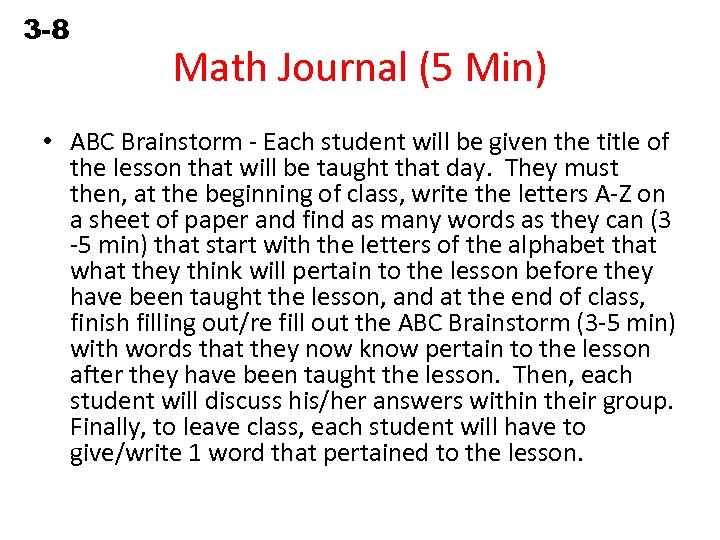3 -8 Solving Equations Containing Fractions Math Journal (5 Min) • ABC Brainstorm - Each student will be given the title of the lesson that will be taught that day. They must then, at the beginning of class, write the letters A-Z on a sheet of paper and find as many words as they can (3 -5 min) that start with the letters of the alphabet that what they think will pertain to the lesson before they have been taught the lesson, and at the end of class, finish filling out/re fill out the ABC Brainstorm (3 -5 min) with words that they now know pertain to the lesson after they have been taught the lesson. Then, each student will discuss his/her answers within their group. Finally, to leave class, each student will have to give/write 1 word that pertained to the lesson.3 -8 Solving Equations Containing Fractions Homework Review (5 Min)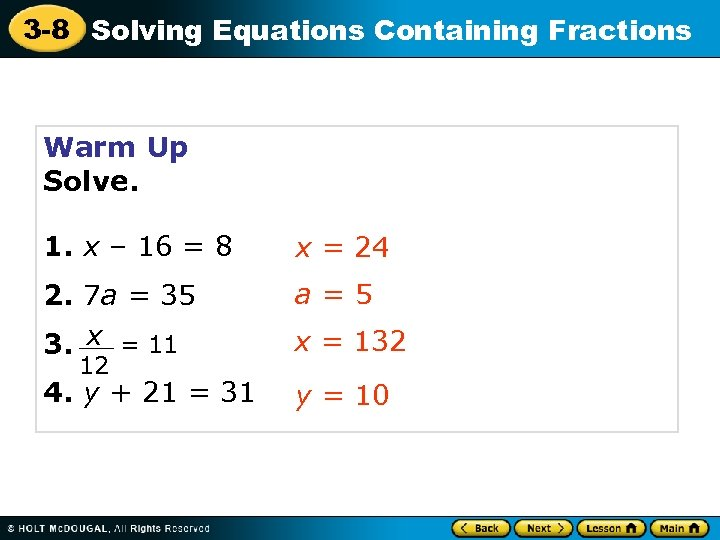3 -8 Solving Equations Containing Fractions Warm Up Solve. 1. x – 16 = 8 x = 24 2. 7 a = 35 3. x = 11 a=5 x = 132 4. y + 21 = 31 y = 10 12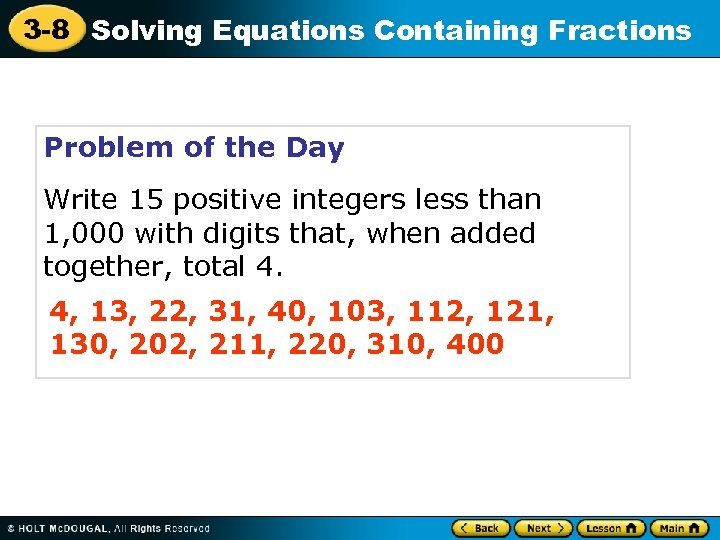3 -8 Solving Equations Containing Fractions Problem of the Day Write 15 positive integers less than 1, 000 with digits that, when added together, total 4. 4, 13, 22, 31, 40, 103, 112, 121, 130, 202, 211, 220, 310, 400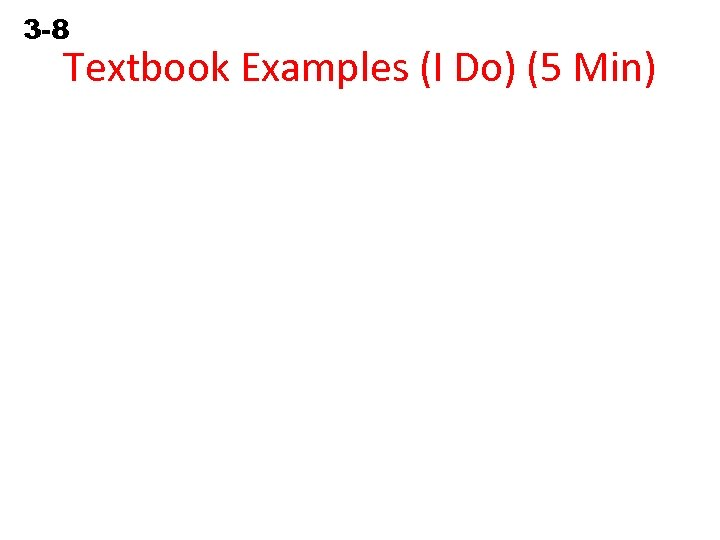3 -8 Solving Equations Containing Fractions Textbook Examples (I Do) (5 Min)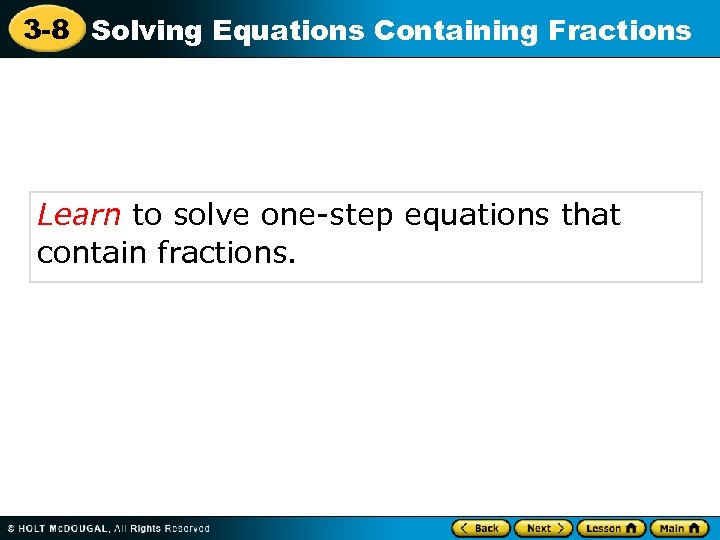3 -8 Solving Equations Containing Fractions Learn to solve one-step equations that contain fractions.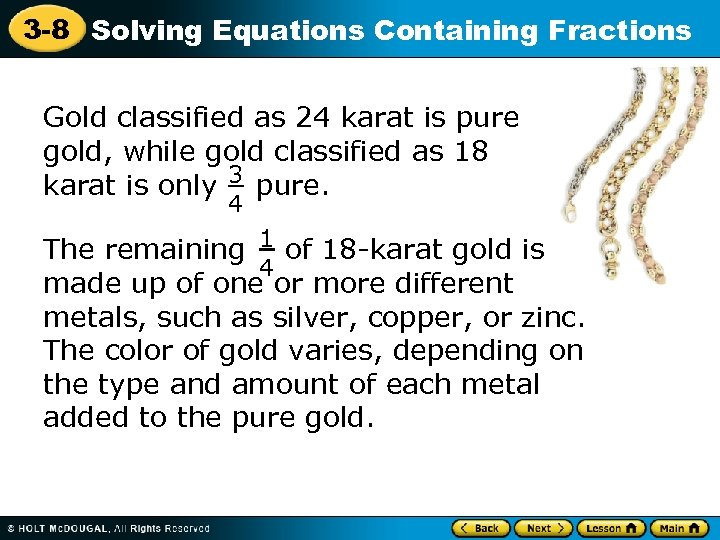3 -8 Solving Equations Containing Fractions Gold classified as 24 karat is pure gold, while gold classified as 18 karat is only 3 pure. 4 The remaining 1 of 18 -karat gold is 4 made up of one or more different metals, such as silver, copper, or zinc. The color of gold varies, depending on the type and amount of each metal added to the pure gold.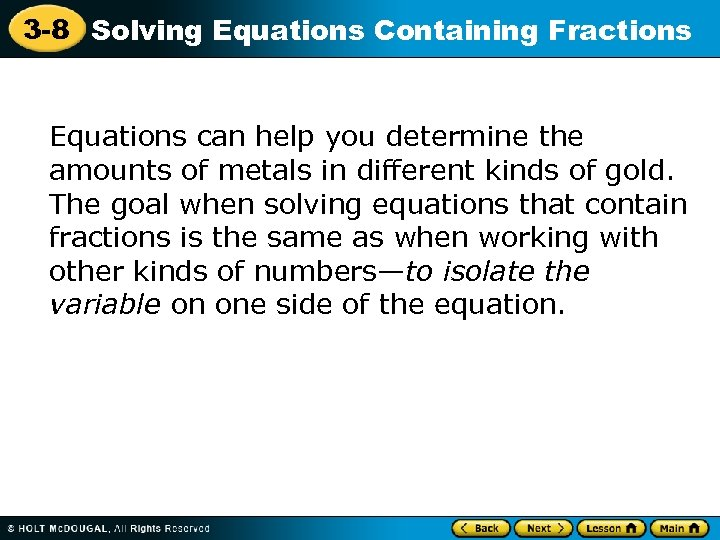3 -8 Solving Equations Containing Fractions Equations can help you determine the amounts of metals in different kinds of gold. The goal when solving equations that contain fractions is the same as when working with other kinds of numbers—to isolate the variable on one side of the equation.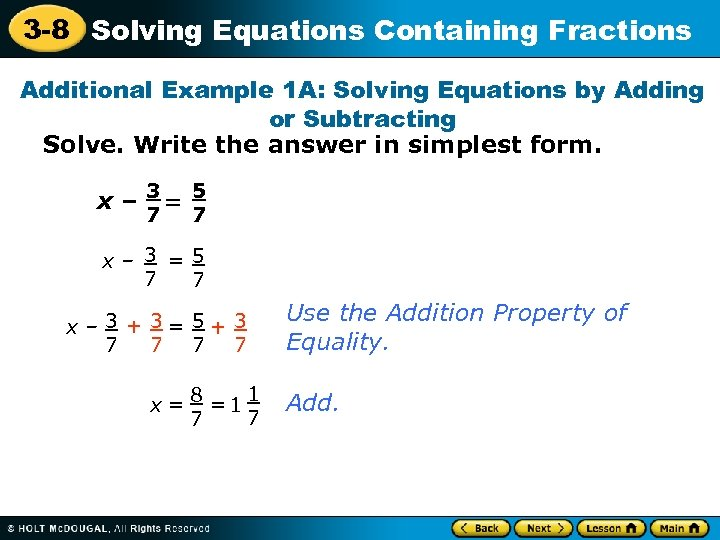3 -8 Solving Equations Containing Fractions Additional Example 1 A: Solving Equations by Adding or Subtracting Solve. Write the answer in simplest form. x – 3= 5 7 7 x– 3 =5 7 7 x – 3 + 3= 5+ 3 7 7 1 x = 8 =1 7 7 Use the Addition Property of Equality. Add.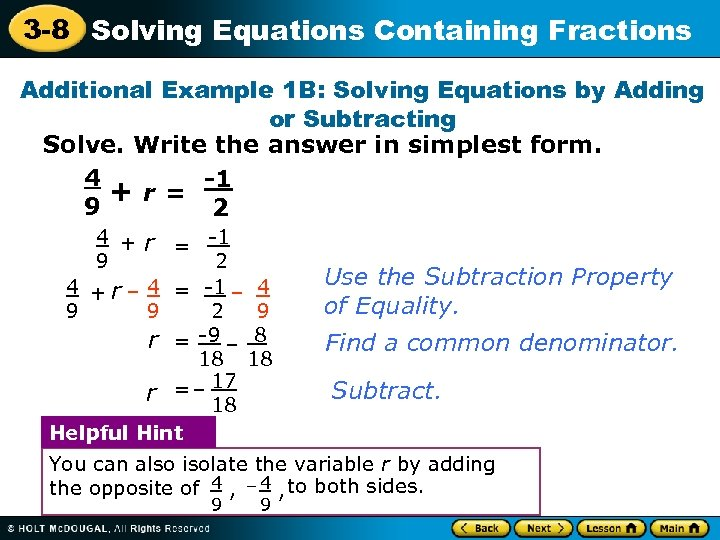3 -8 Solving Equations Containing Fractions Additional Example 1 B: Solving Equations by Adding or Subtracting Solve. Write the answer in simplest form. 4 -1 +r= 9 2 4 + r = -1 9 2 Use the Subtraction Property 4 + r – 4 = -1 – 4 of Equality. 2 9 9 9 r = -9 – 8 Find a common denominator. 18 18 17 r = – 18 Helpful Hint Subtract. You can also isolate the variable r by adding the opposite of 4 , – 4 , to both sides. 9 9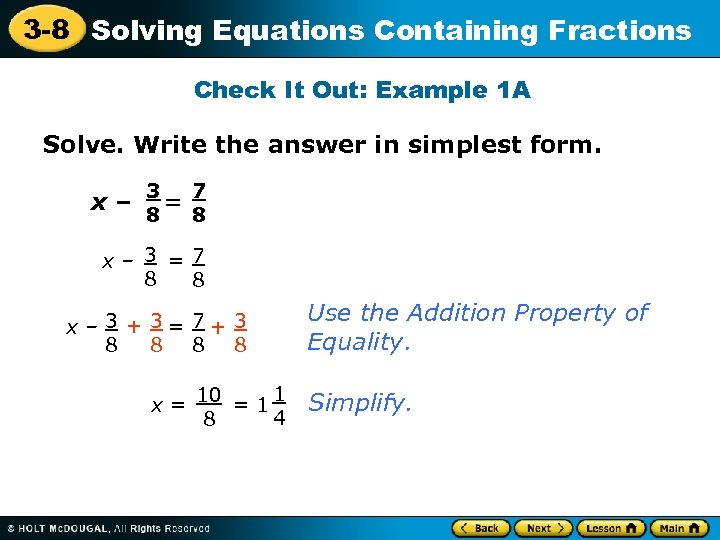3 -8 Solving Equations Containing Fractions Check It Out: Example 1 A Solve. Write the answer in simplest form. x – 3= 7 8 8 x– 3 =7 8 8 x – 3 + 3= 7+ 3 8 8 1 x = 10 = 1 4 8 Use the Addition Property of Equality. Simplify.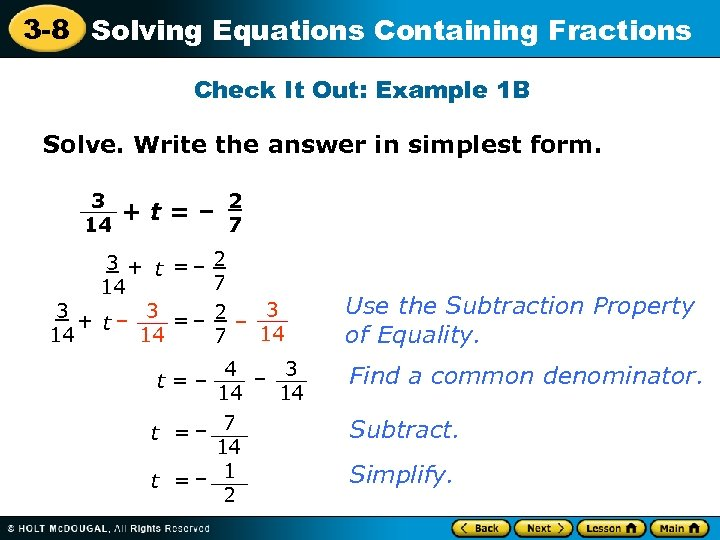3 -8 Solving Equations Containing Fractions Check It Out: Example 1 B Solve. Write the answer in simplest form. 3 14 +t=– 2 7 3 + t =– 2 7 14 3 3 3 + t– =– 2 – 14 14 14 7 4 – 3 t=– 14 14 7 t =– 14 1 t =– 2 Use the Subtraction Property of Equality. Find a common denominator. Subtract. Simplify.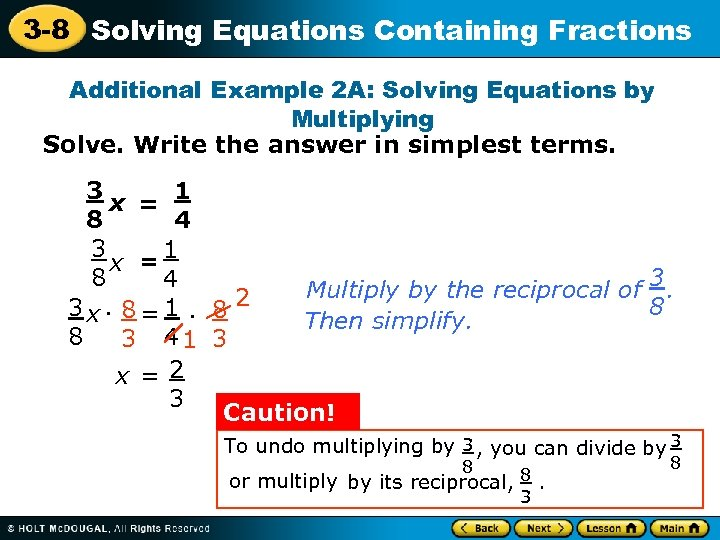3 -8 Solving Equations Containing Fractions Additional Example 2 A: Solving Equations by Multiplying Solve. Write the answer in simplest terms. 3 x 1 = 8 4 3 1 x = 8 4 Multiply by the reciprocal of 3. 8 3 x. 8= 1. 8 2 Then simplify. 8 3 41 3 x =2 3 Caution! To undo multiplying by 3 , you can divide by 3 8 or multiply by its reciprocal, 8. 3 8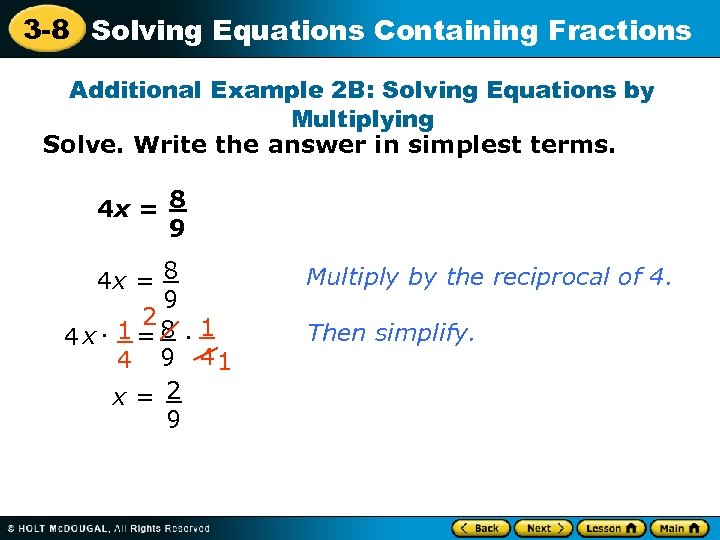3 -8 Solving Equations Containing Fractions Additional Example 2 B: Solving Equations by Multiplying Solve. Write the answer in simplest terms. 4 x = 8 9 2. 1= 8. 1 4 x 4 9 41 x= 2 9 Multiply by the reciprocal of 4. Then simplify.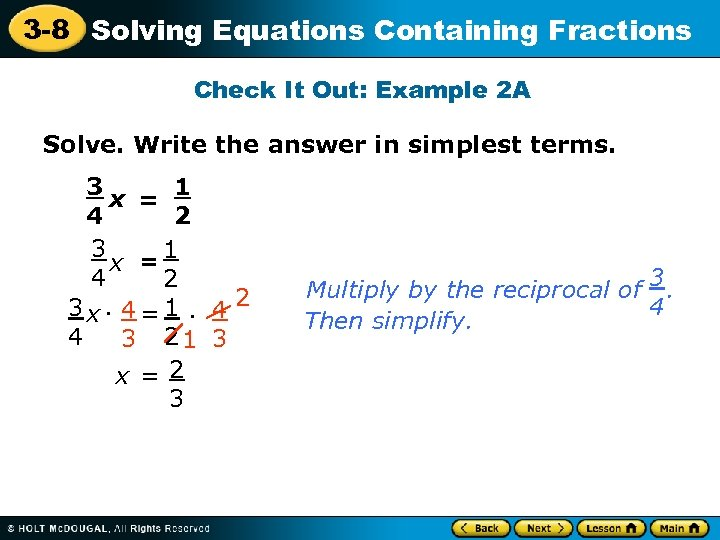3 -8 Solving Equations Containing Fractions Check It Out: Example 2 A Solve. Write the answer in simplest terms. 3 x 1 = 4 2 3 1 x = 4 2 3 x. 4= 1. 4 2 4 3 21 3 x =2 3 Multiply by the reciprocal of 3. 4 Then simplify.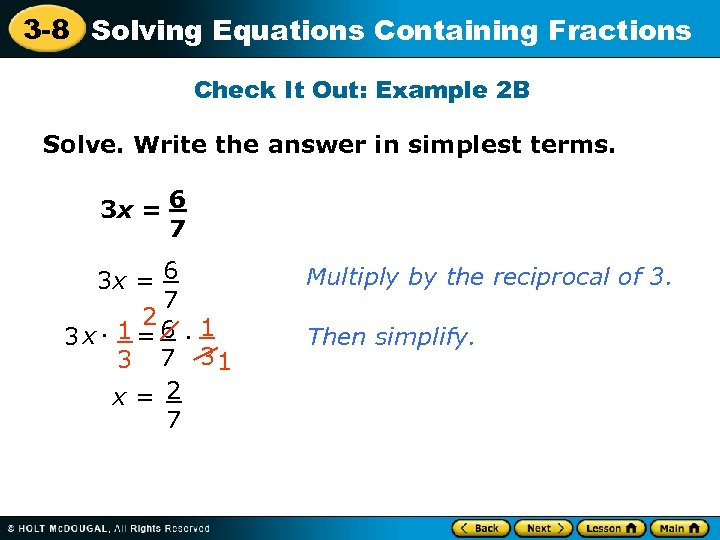3 -8 Solving Equations Containing Fractions Check It Out: Example 2 B Solve. Write the answer in simplest terms. 3 x = 6 7 2. 1= 6. 1 3 x 3 7 31 x= 2 7 Multiply by the reciprocal of 3. Then simplify.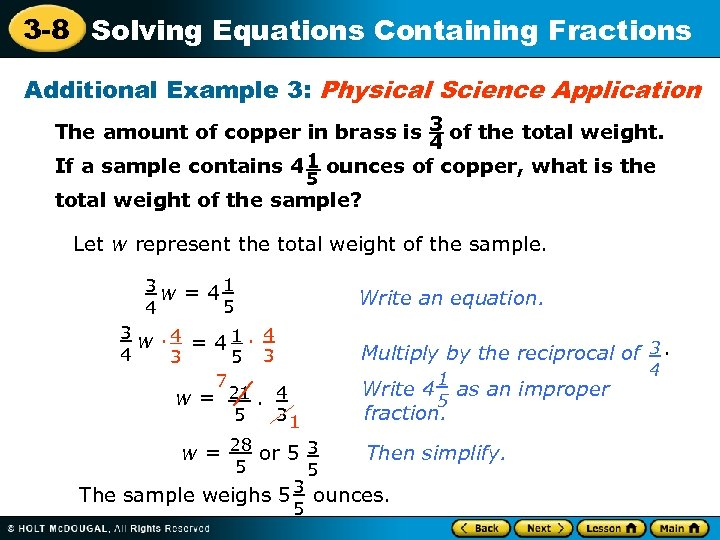3 -8 Solving Equations Containing Fractions Additional Example 3: Physical Science Application The amount of copper in brass is 3 of the total weight. 4 If a sample contains 4 1 ounces of copper, what is the 5 total weight of the sample? Let w represent the total weight of the sample. 3 w = 41 5 4 Write an equation. 3 w · 4 = 41· 4 4 3 5 3 7 w = 21 · 4 5 31 Multiply by the reciprocal of 3 · 1 Write 4 as an improper 5 fraction. w = 28 or 5 3 5 5 Then simplify. The sample weighs 5 3 ounces. 5 4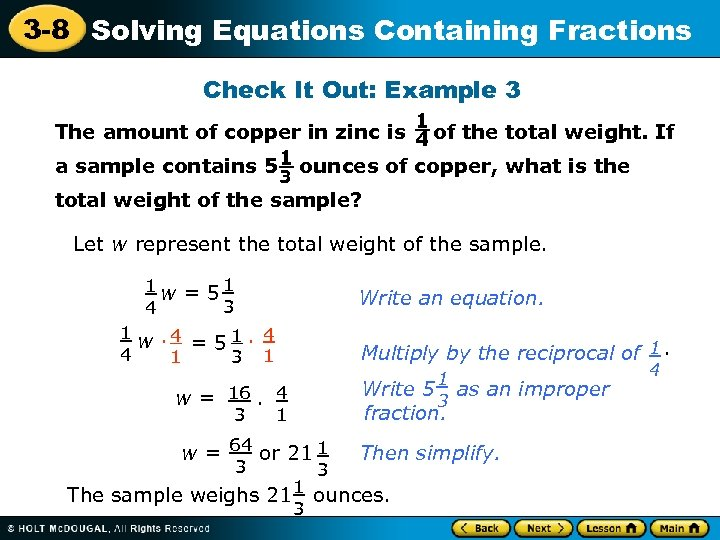3 -8 Solving Equations Containing Fractions Check It Out: Example 3 1 The amount of copper in zinc is 4 of the total weight. If a sample contains 5 1 ounces of copper, what is the 3 total weight of the sample? Let w represent the total weight of the sample. 1 w = 51 3 4 Write an equation. 1 w · 4 = 51· 4 4 1 3 1 Multiply by the reciprocal of 1 · 1 Write 5 as an improper 3 w = 16 · 4 3 fraction. 1 w = 64 or 21 1 3 3 Then simplify. The sample weighs 21 1 ounces. 3 4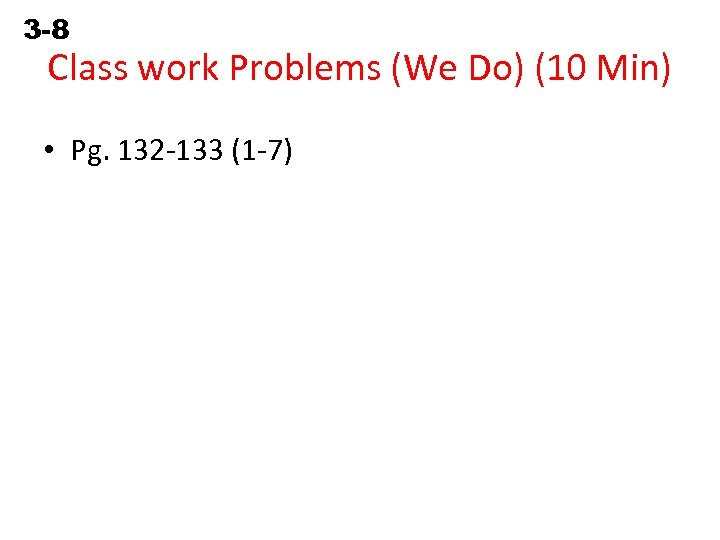3 -8 Solving Equations Containing Fractions Class work Problems (We Do) (10 Min) • Pg. 132 -133 (1 -7)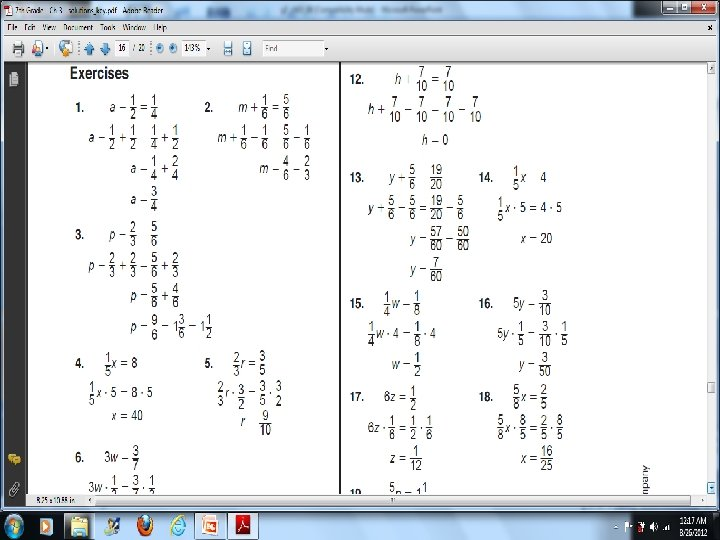3 -8 Solving Equations Containing Fractions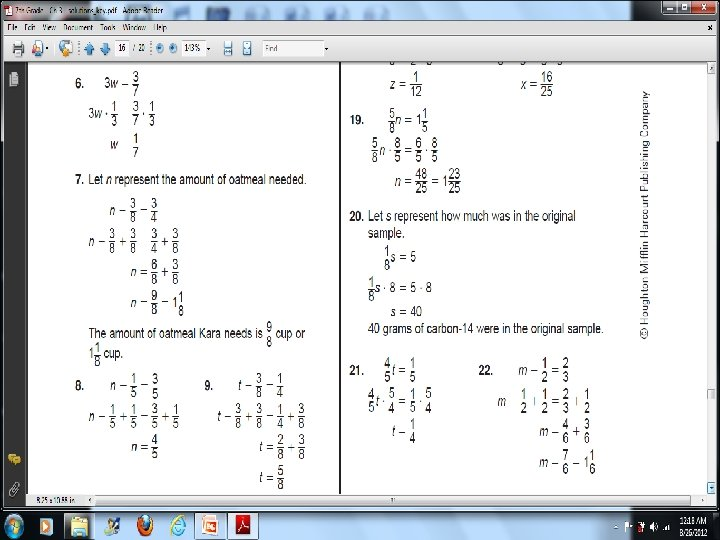3 -8 Solving Equations Containing Fractions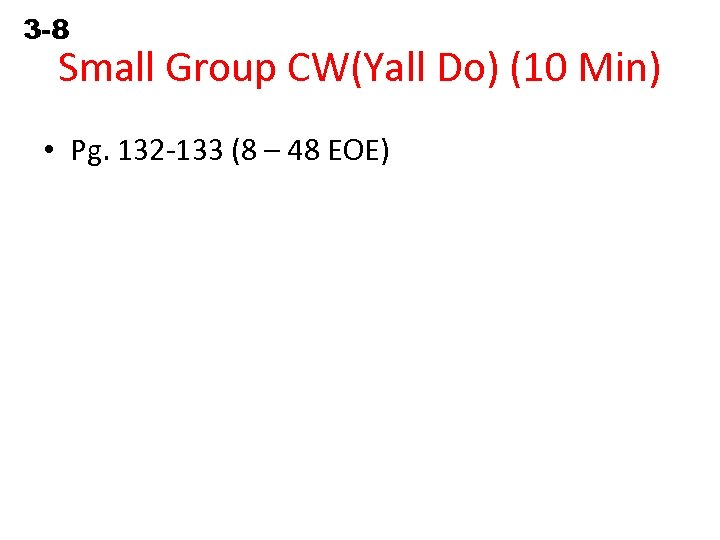3 -8 Solving Equations Containing Fractions Small Group CW(Yall Do) (10 Min) • Pg. 132 -133 (8 – 48 EOE)3 -8 Solving Equations Containing Fractions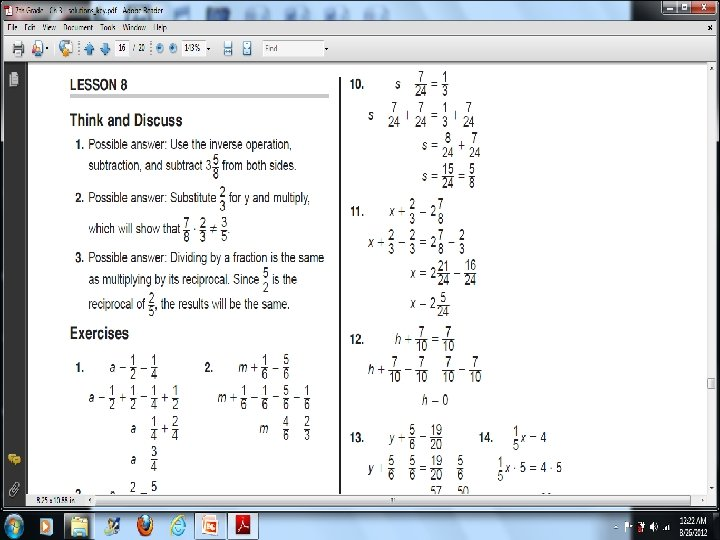3 -8 Solving Equations Containing Fractions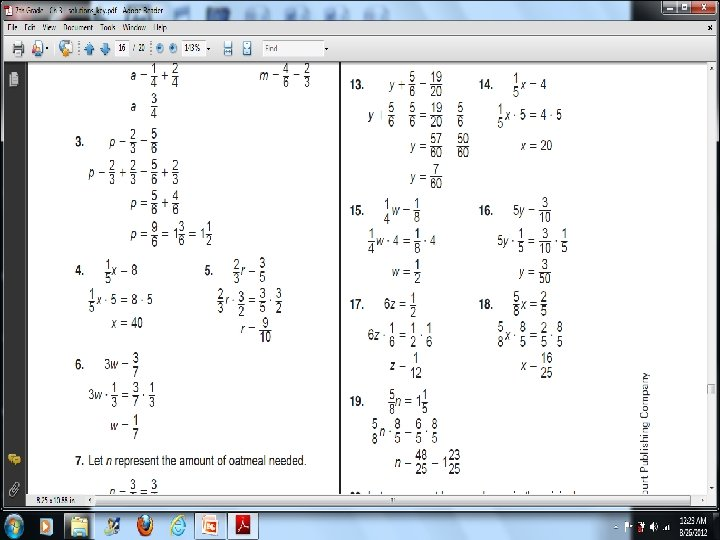3 -8 Solving Equations Containing Fractions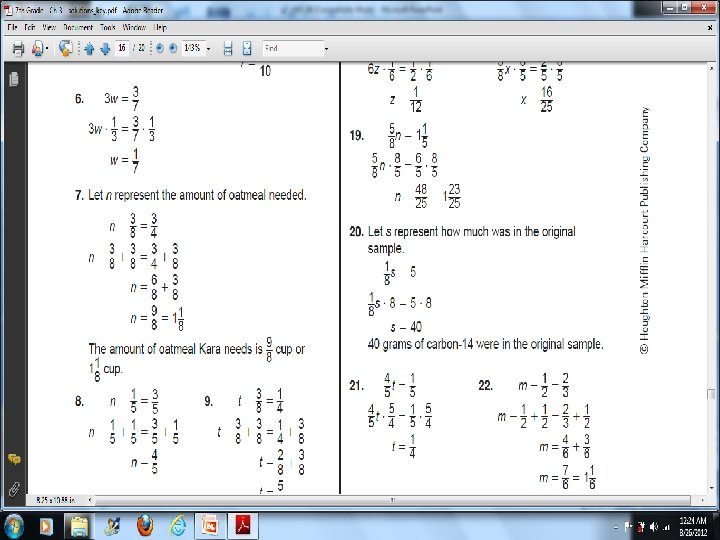3 -8 Solving Equations Containing Fractions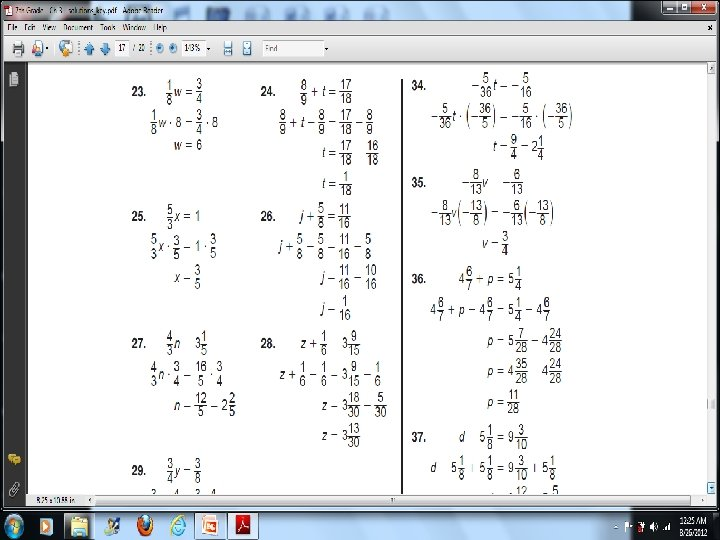3 -8 Solving Equations Containing Fractions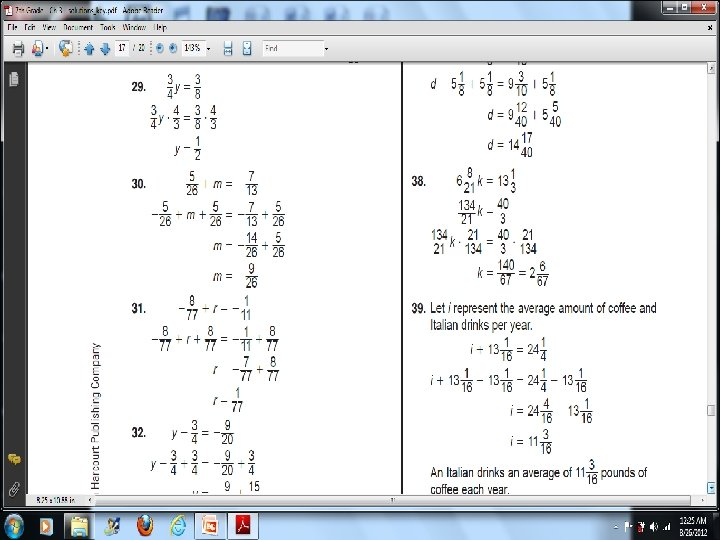3 -8 Solving Equations Containing Fractions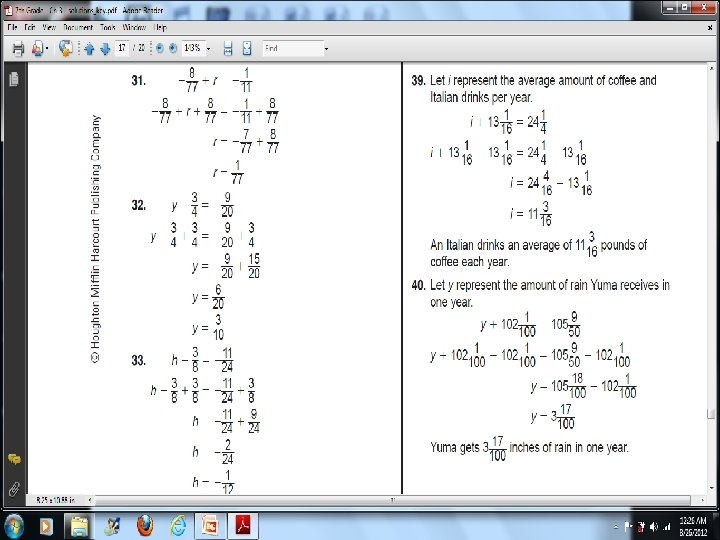3 -8 Solving Equations Containing Fractions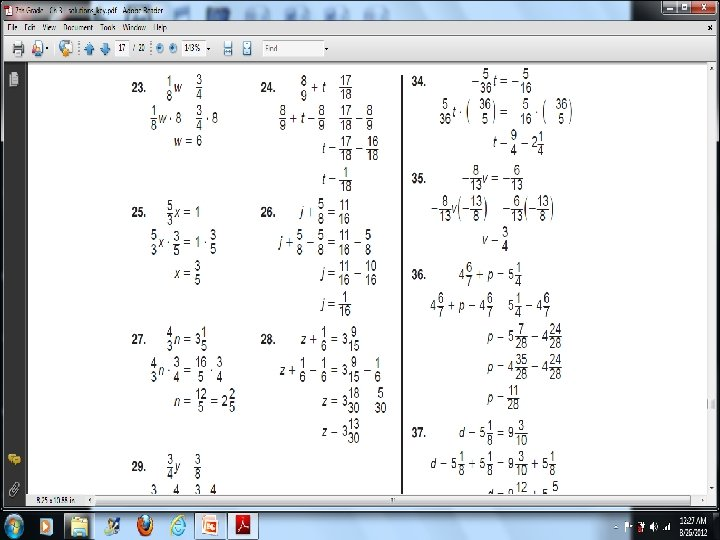3 -8 Solving Equations Containing Fractions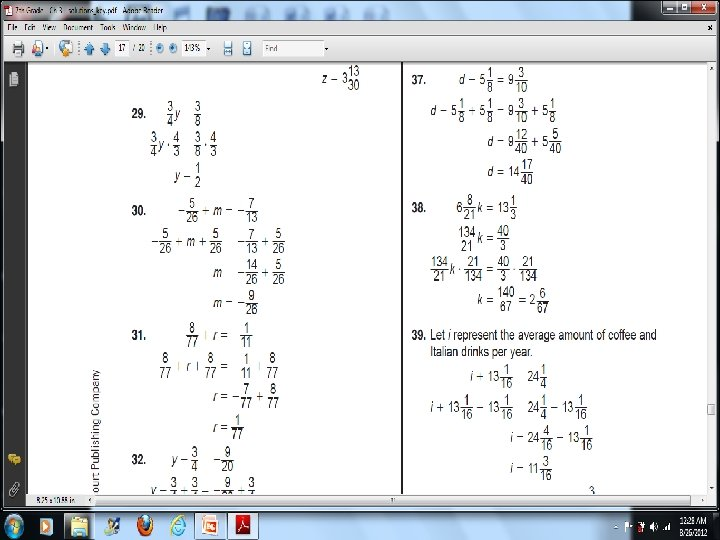3 -8 Solving Equations Containing Fractions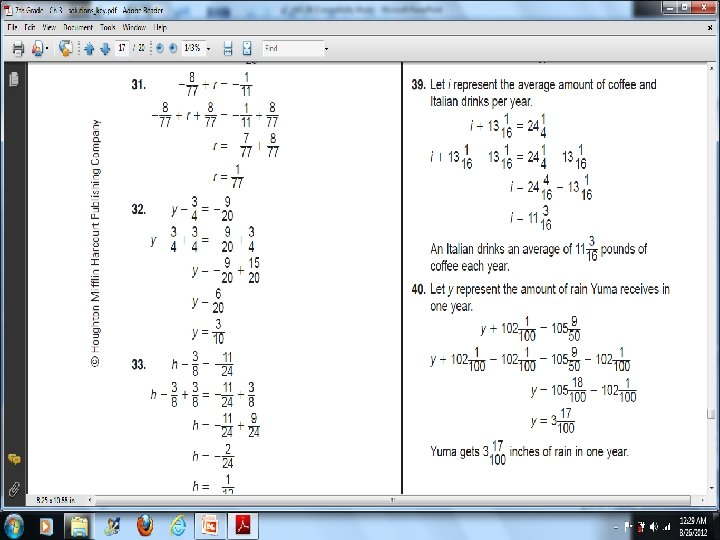3 -8 Solving Equations Containing Fractions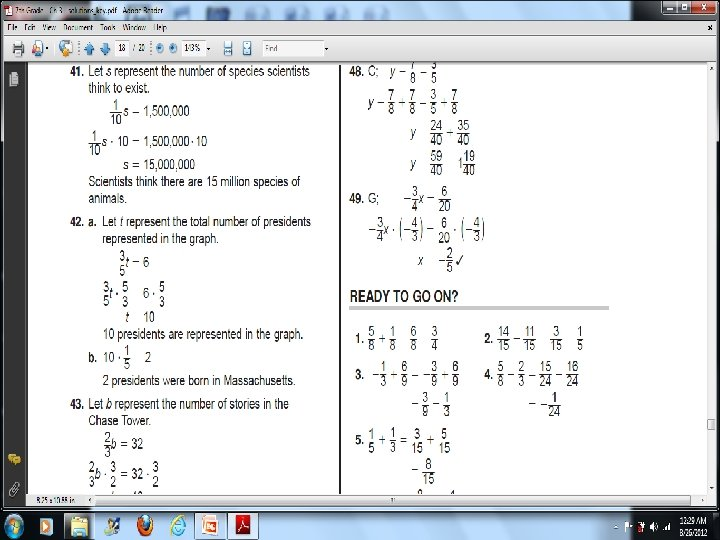3 -8 Solving Equations Containing Fractions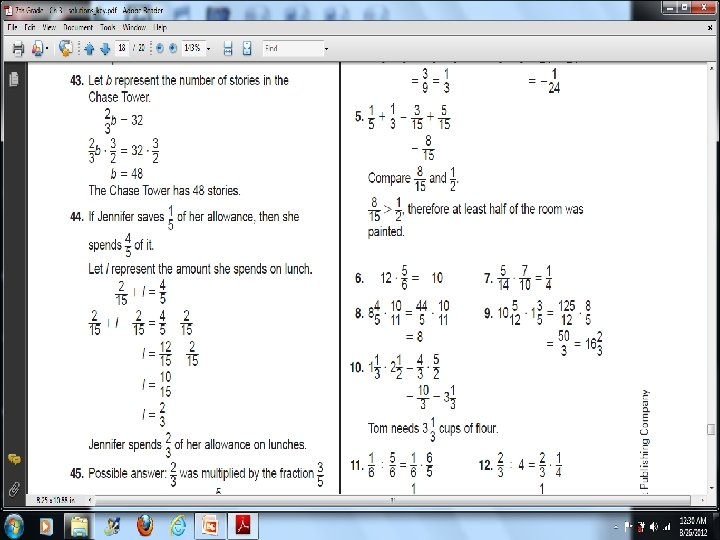3 -8 Solving Equations Containing Fractions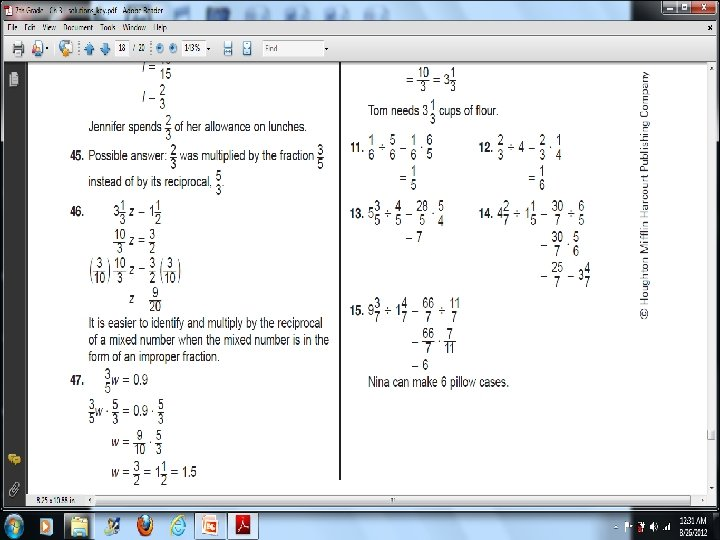3 -8 Solving Equations Containing Fractions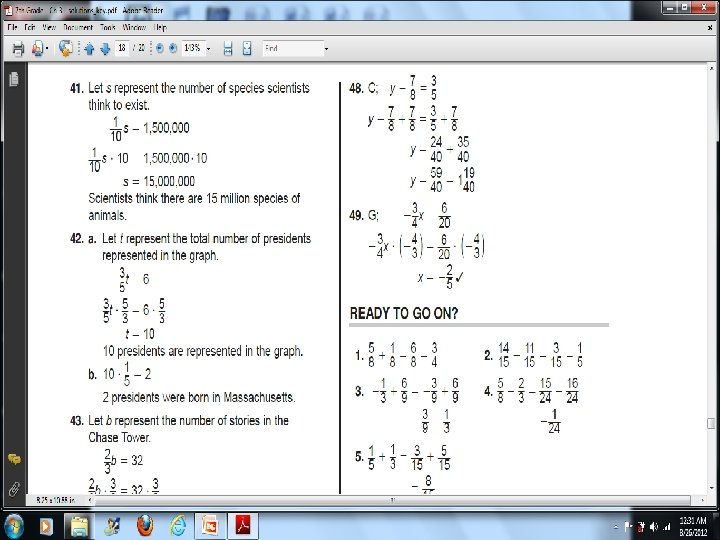3 -8 Solving Equations Containing Fractions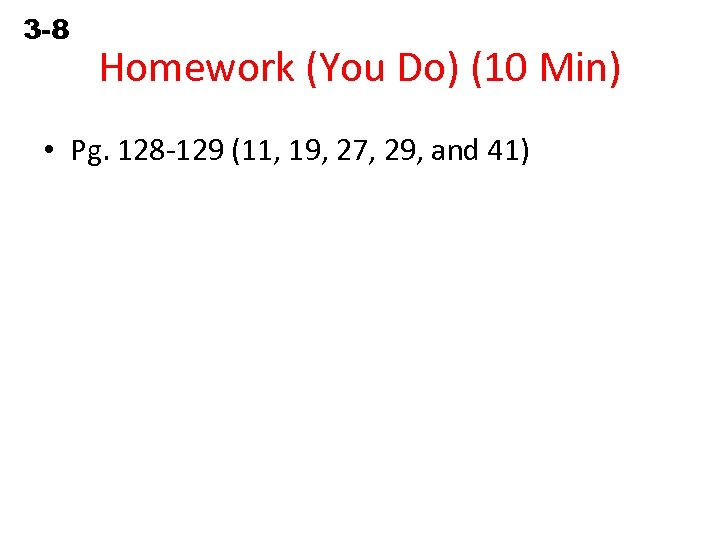3 -8 Solving Equations Containing Fractions Homework (You Do) (10 Min) • Pg. 128 -129 (11, 19, 27, 29, and 41)3 -8 Solving Equations Containing Fractions Math Journal (5 Min) • ABC Brainstorm - Each student will be given the title of the lesson that will be taught that day. They must then, at the beginning of class, write the letters A-Z on a sheet of paper and find as many words as they can (3 -5 min) that start with the letters of the alphabet that what they think will pertain to the lesson before they have been taught the lesson, and at the end of class, finish filling out/re fill out the ABC Brainstorm (3 -5 min) with words that they now know pertain to the lesson after they have been taught the lesson. Then, each student will discuss his/her answers within their group. Finally, to leave class, each student will have to give/write 1 word that pertained to the lesson.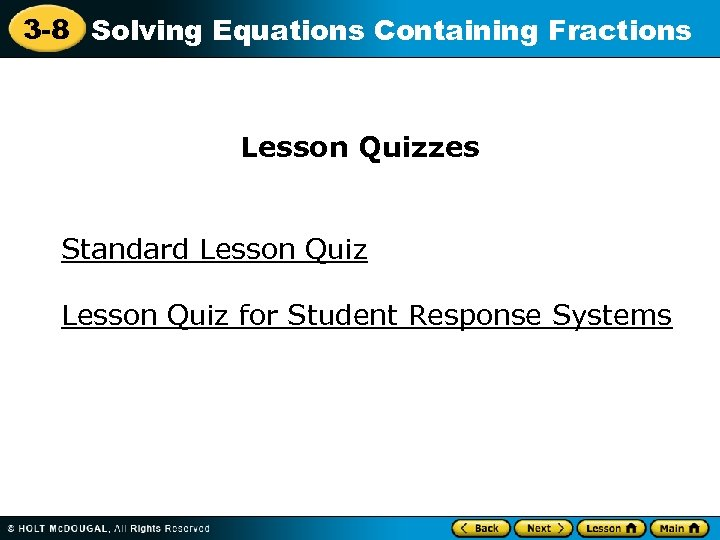3 -8 Solving Equations Containing Fractions Lesson Quizzes Standard Lesson Quiz for Student Response Systems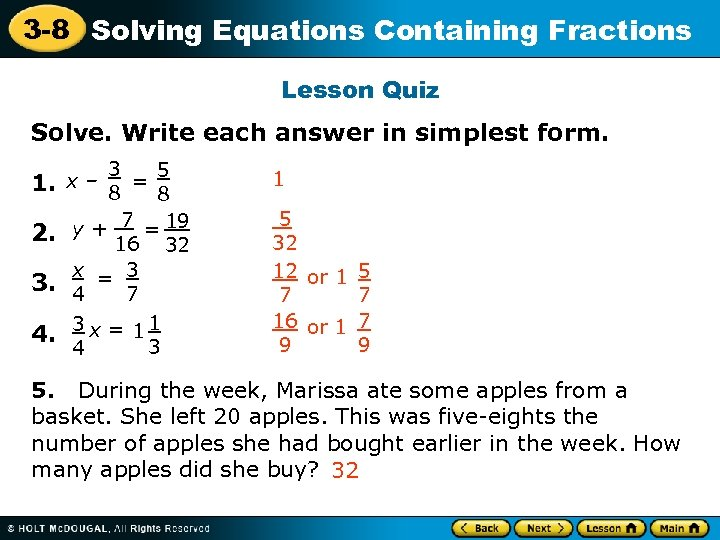3 -8 Solving Equations Containing Fractions Lesson Quiz Solve. Write each answer in simplest form. 3 5 1. x – 8 = 8 2. 3. 4. y + 7 = 19 16 32 x = 3 4 7 3 x = 11 3 4 1 5 32 12 or 1 5 7 7 16 or 1 7 9 9 5. During the week, Marissa ate some apples from a basket. She left 20 apples. This was five-eights the number of apples she had bought earlier in the week. How many apples did she buy? 32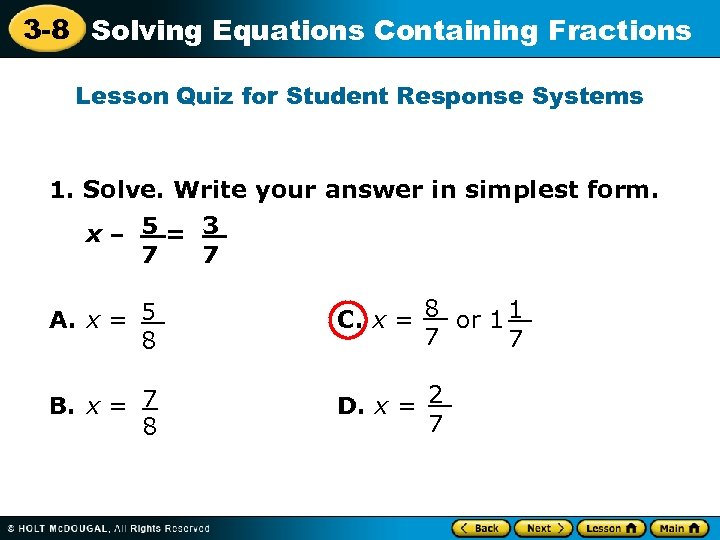3 -8 Solving Equations Containing Fractions Lesson Quiz for Student Response Systems 1. Solve. Write your answer in simplest form. x– 5= 3 7 7 A. x = 5 8 C. x = 8 or 1 1 7 7 B. x = 7 8 D. x = 2 7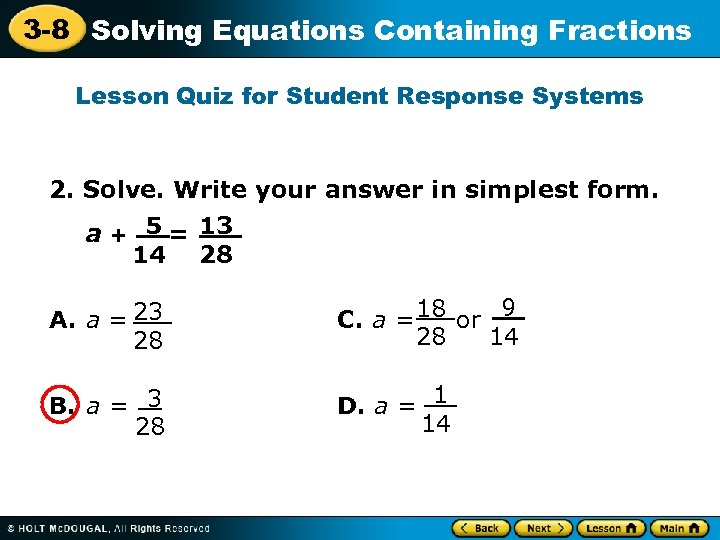3 -8 Solving Equations Containing Fractions Lesson Quiz for Student Response Systems 2. Solve. Write your answer in simplest form. a + 5 = 13 14 28 A. a = 23 28 C. a = 18 or 9 14 28 B. a = 3 28 D. a = 1 14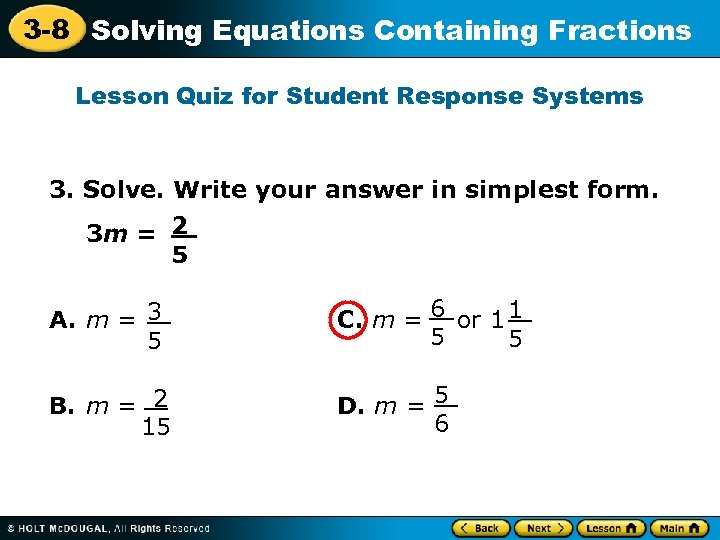3 -8 Solving Equations Containing Fractions Lesson Quiz for Student Response Systems 3. Solve. Write your answer in simplest form. 3 m = 2 5 A. m = 3 5 C. m = 6 or 1 1 5 5 B. m = 2 15 D. m = 5 6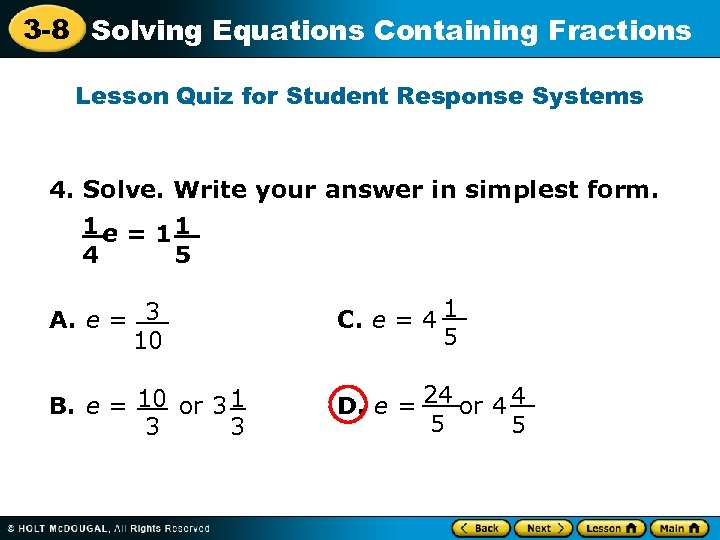3 -8 Solving Equations Containing Fractions Lesson Quiz for Student Response Systems 4. Solve. Write your answer in simplest form. 1 e = 11 4 5 A. e = 3 10 C. e = 4 1 5 B. e = 10 or 3 1 3 3 D. e = 24 or 4 4 5 5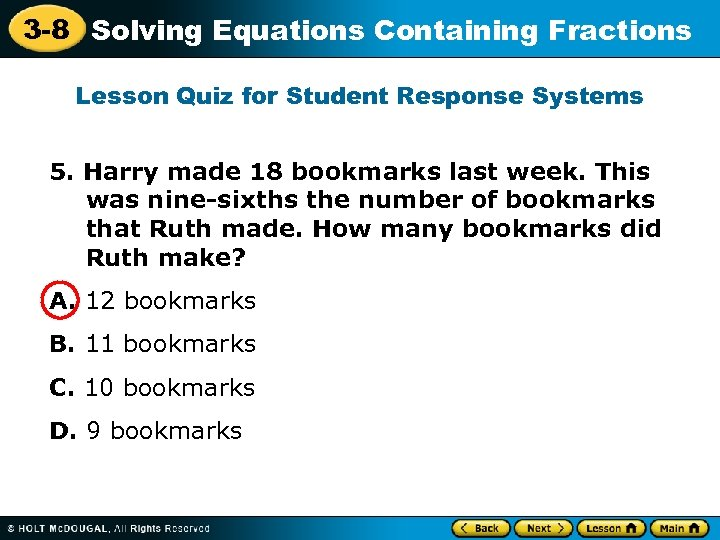3 -8 Solving Equations Containing Fractions Lesson Quiz for Student Response Systems 5. Harry made 18 bookmarks last week. This was nine-sixths the number of bookmarks that Ruth made. How many bookmarks did Ruth make? A. 12 bookmarks B. 11 bookmarks C. 10 bookmarks D. 9 bookmarks